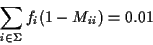Next: Phylogenetic tree. Up: Definitions Previous: Mutation model.

#### PAM distance.

In the definition of mutation, we use the matrix M which implies certain amount of mutation. For all our work it is important to quantify mutation. We will use the terminology introduced by Dayhoff et al.  where amount of mutation is measured in PAM units.

A 1-PAM mutation matrix describes an amount of evolution which will change, on the average, 1% of the amino acids. In mathematical terms this is expressed as a matrix M such thatThe diagonal elements of M are the probabilities that a given amino acid does not change, so (1-Mii) is the probability of mutating away from i.

If we have a probability or frequency vector p, the product Mp gives the probability vector or the expected frequency of p after an evolution equivalent to 1-PAM unit. Or, if we start with amino acid i (a probability vector which contains a 1 in position i and 0s in all others) M*i (the ith column of M) is the corresponding probability vector after one unit of random evolution. Similarly, after k units of evolution (what is called k-PAM evolution) a frequency vector p will be changed into the frequency vector Mk p. Notice that chronological time is not linearly dependent on PAM distance. Evolution rates may be very different for different species and different proteins.Next: Phylogenetic tree. Up: Definitions Previous: Mutation model.
Gaston Gonnet
1998-07-14